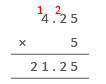## Multiplying Decimals with Whole Number

Practice Unlimited Questions

#### How to Multiply Decimals with Whole Number?

Step 1: Multiply the hundredths.

Step 2: Multiply and add the tenths.

Step 3: Multiply and add the ones.

See working examples below.

#### 1. Bob had 3 packets of butter each containing 0.4 kg butter. How much butter did Bob have altogether?

Method 1:
0.4  +  0.4  +  0.4  =  1.2 kg
Bob had 1.2 kg of butter altogether.

Method 2:
Multiply 0.4 kg by 3.
0.4  ×  3  =  ?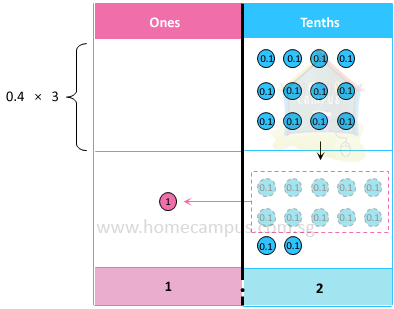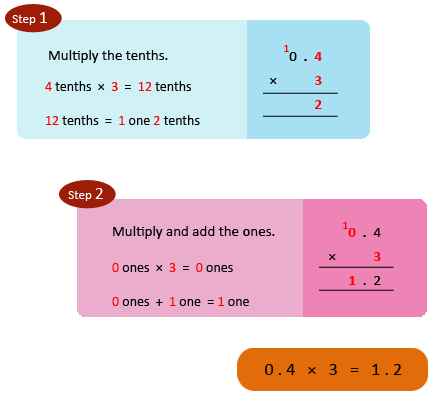Bob had 1.2 kg of butter altogether.

#### 2. Bob had 3 cartons of milk each containing 0.45 L of milk. How much milk did Bob have altogether?

Multiply 0.45 L by 3.
0.45  ×  3  =  ?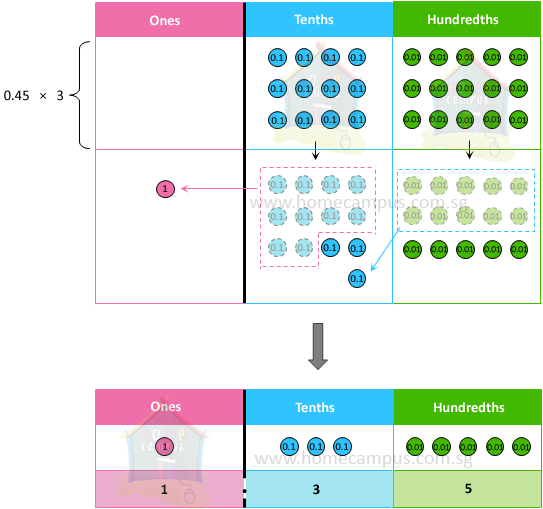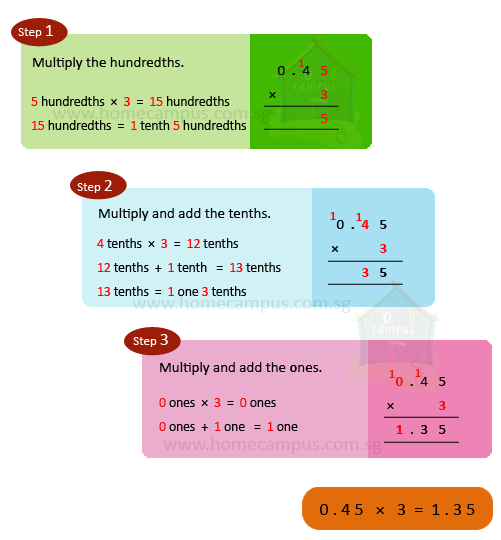Bob had 1.35 L of milk altogether.

#### 3. Bob had 7 bags of flour each containing 1.85 kg flour. How much flour did Bob have altogether?

Multiply 1.85 kg by 7.
1.85  ×  7  =  ?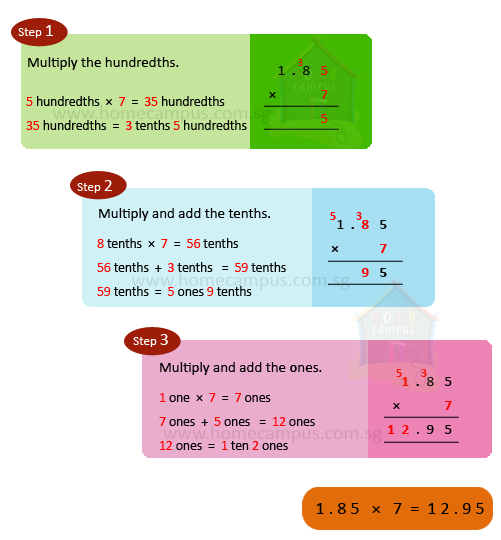Bob had 12.95 kg of flour altogether.

#### 4. Serena bought 5 drawing books for \$4.25 each. How much did she spend on the drawing books altogether?

Cost of 1 drawing book   =   \$4.25
Cost of 5 drawing books   =   \$4.25  ×  5
=   \$21.25

She spent \$21.25 on the drawing books altogether.